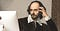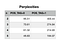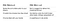# Abstract

This experiment considers the effect of using part-of-speech tags instead of words to create an n-gram model from a corpus. The goal of using part-of-speech tags is to mitigate the sparsity that comes from higher-order word n-gram models. Shakespeare’s plays were used as training and test corpora. After using part-of-speech tag n-grams to calculate log probabilities on the test corpora, word probabilities are incorporated by using the MLE estimates for P(word|tag). The perplexities of using a word n-gram model and a part-of-speech tag n-gram model are compared to find that the part-of-speech model severely underperforms. See the discussion for an analysis.

Technologies and data used: Python, NLTK, UNIX shell scripting, ibiblio for Shakespeare corporaHow he probably was bein’

# Introduction

One of the most basic ways to start generating natural language is by counting up the frequencies of words in different contexts. For example, if you notice that the word “party” comes after “surprise” fairly often, then in your own speech, you could start following “surprise” with “party” to make yourself sound natural.

Computers are well-suited for this task. They can read in huge chunks of text and count up how many times words, pairs of words, three-tuples of words, etc. occur together. Then, they can use those counts to create probabilities of words and sentences.

Consider this example:

The word “relish” happens three times in our corpus (fancy word for chunk of text), once with “swimming” after it and twice with “traveling” after it. So, we can say that the probability of “swimming” occurring after “traveling” is 1/3. Naturally, the probability of “traveling” after “relish” is 2/3.

More specifically, the probability of word w given word v is the count of how many times w occurs after v, divided by how many times v occurs.

Generalizing, we consider the probability of a word w given the preceding words x1, x2, … x(n-1) where n is a positive integer. This probability is the frequency of the sequence (x1, x2 … x(n-1), w) over the frequency of the sequence (x1, x2, … x(n-1)). These basic probabilities comprise an n-gram model. The sequence containing w is an n-gram since it has n words, and the sequence without it is an (n-1)-gram.

Often, n cannot be greater than 5 because you fall prey to overfitting or data sparsity. In terms of data sparsity, you might run into 4-grams or 5-grams in your test data that you never saw in your training data. Your count for these higher-order grams is 0, and so their probability is 0.

There are several probability smoothing techniques used to handle these zero-probability cases––the ones I used below are: add-k smoothing, linear interpolation, and Katz’ Backoff (see section 4.5 in linked textbook chapter). But I was hoping to find a way to densify the training data to improve performance with n-gram language models.

This experiment considers using part-of-speech tags instead of words themselves to construct an n-gram model. Since the number of part-of-speech tags is far fewer than the number of words in a corpus, switching from one token set to the other greatly densifies the data. Then, we use the conditional probabilities of a word given a part-of-speech tag––calculated in a similar way as above with maximum-likelihood learning––to bring the words themselves back into the model.

# Set-Up

I wrote an n-gram model in Python and an evaluation script to see if I could achieve a lower perplexity using part-of-speech tags in the n-grams instead of words. All source code can be found here.

The Python class Ngram_model takes in the following parameters:

• a training corpus from which to extract the counts and probabilities
• an integer n, the size of n-grams for this instance of the model
• an integer POS_TAG, where POS_TAG = 0 if the model should use part-of-speech tags to create ngrams, and POS_TAG = 1 if the model should use words
• a string smoother, where smoother=”interpolate” or smoother=”backoff” to denote which method to smooth n-gram probabilities

The model uses NLTK to tokenize and tag the corpus. The corpora for testing and training are formatted through a UNIX shell script with simple grep and sed commands.

The evaluation script then creates instances of the model on the same training corpus using words for n-grams and POS-tags for n-grams for each n between 2 and 5. It calculates the perplexity of these models on the same test corpus.

I separated Shakespeare’s plays into training, tuning, and test data following a rough 80–10–10 split. I kept a balance of comedies, tragedies, and histories in each category using my own human knowledge. Anybody who would like to use the source code to try the experiment themselves can change the split how they see fit.

In terms of smoothing, I resorted to linear interpolation, while leaving functionality in the source code for more advanced practices. I was working with a personal MacBook Pro, so my computational capacity was limited. Unfortunately, running the recursive Katz’ Backoff on the language models that used words for the n-grams took much too long. In terms of parameter tuning for both Katz’ Backoff and linear interpolation, the functionality is there in the code, but I did not use it for the same reason. I leave it for future work to play around with tuning.

# Results and DiscussionPerplexity values on the same test corpus for different instances of Ngram_model with parameters as shown.

At a glance, it’s obvious that the word n-gram models perform far better than the part-of-speech ones do. An important note is that the M-value I used in calculating perplexity (i.e. the value you use to take the M-th root of the product of all the probabilities) is the same among instances of the model that have POS_TAG=0 and POS_TAG=1.

I made this choice based on an interpretation. When POS_TAG=0, we have an n-gram’s probability represented by P(word|prev_words). When POS_TAG=1, I interpret the n-gram’s probability as represented by P(word|tag) * P(tag|prev_tags). You could argue that because P(tag|prev_tags)*P(word|tag) are separate probabilities, the M value when POS_TAG=1 should be double than when POS_TAG=0. I disagree in this instance, because we are specifically doing a comparison between these two models. Regardless, this choice is part of the reason the models with POS_TAG=1 underperform so greatly.Sample sentences generated by a part-of-speech model (left) and a word model (right).

The part-of-speech models don’t just do poorly mathematically, either. The sentences generated by the word models are much more natural than those of the part-of-speech models, based on my personal subjective analysis. What are the fundamental reasons behind why the POS models fare so badly? A likely reason is that a relatively high-order n-gram of part-of-speech tags does not encode any new information that an n-gram of words does. This is because given three or four words in sequence, it’s not often ambiguous what the parts of speeches of the words are in this context.

Another issue is that the words are now completely taken out of context, so that they only depend on their surrounding words syntactically but not semantically. Future study could investigate whether using very high-order part-of-speech tags for n-grams while using 3- or 4-grams of words in tandem could have a greater effect.